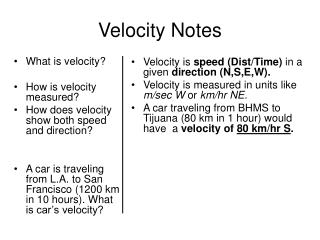# Velocity Notes - PowerPoint PPT PresentationDownload PresentationVelocity Notes

Velocity NotesDownload Presentation## Velocity Notes

- - - - - - - - - - - - - - - - - - - - - - - - - - - E N D - - - - - - - - - - - - - - - - - - - - - - - - - - -
##### Presentation Transcript

1. What is velocity? How is velocity measured? How does velocity show both speed and direction? A car is traveling from L.A. to San Francisco (1200 km in 10 hours). What is car’s velocity? Velocity is speed (Dist/Time) in a given direction (N,S,E,W). Velocity is measured in units like m/sec W or km/hr NE. A car traveling from BHMS to Tijuana (80 km in 1 hour) would have a velocity of 80 km/hr S. Velocity Notes

2. How are velocities combined? (draw picture) If two velocities are in the same direction they are added together. WIND = 10 km/hr West Total Velocity = 15 km/hr West Current = 5 km/hr West

3. How are velocities in opposite directions combined? (draw picture) If velocities are in the opposite direction they are subtracted. WIND = 10 km/hr West Total Velocity = 5 km/hr West Current = 5 km/hr East

4. Why do rockets take off in the same direction as the earth’s rotation? Answer this Question

5. Why does it take less time to fly from California to New York than to go from NY to California? Answer this Question JET STREAM

6. Summary • Write the shortest summary you can that uses the following words: • Velocity, speed, direction, km/hr East, same, opposite, add, subtract,.